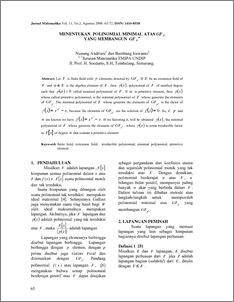# MENENTUKAN POLINOMIAL MINIMAL ATAS GF p YANG MEMBANGUN GF p n

Andriani, Nunung and Irawanto, Bambang (2008) MENENTUKAN POLINOMIAL MINIMAL ATAS GF p YANG MEMBANGUN GF p n. JURNAL MATEMATIKA DAN KOMPUTER, 11 (2). pp. 65-72. ISSN 1510-8518Preview
PDF
162Kb

## Abstract

Let is finite field with elements, denoted by . If be an extension field of and is the algebra element of , then , polynomial of of smallest degree such that called minimal polynomial of . If is primitive element, then whose called primitive polynomial, is the minimal polynomial of whose generate the elements of .The minimal polynomial of whose generate the elements of is the factor of , because the elements of are the solution of . So, if and are known we have . If we factoring it, will be obtained , the minimal polynomial of whose generate the elements of , where is some irreducible factor in of degree that contain a primitive element.

Item Type: Article finite field, extension field, irreducible polynomial, minimal polynomial, primitive element. Q Science > QA Mathematics Faculty of Science and Mathematics > Department of Mathematics 1946 INVALID USER 01 Dec 2009 00:44 04 Dec 2009 08:38

Repository Staff Only: item control page